TUTORIALS
TUTORIALS HOME

GENERAL MATH
NOTATION & METHODS OF PROOF
INDUCTION
COMPLEX NUMBERS
POLYNOMIALS

LINEAR ALGEBRA
VECTORS
SYSTEM OF LINEAR EQUATIONS
MATRICES
EIGENVALUES & EIGENVECTORS
ORTHOGONALITY
VECTOR SPACE
DISTANCE & APPROXIMATION

HOME
TESTS
TUTORIALS
SAMPLE PROBLEMS
COMMON MISTAKES
STUDY TIPS
GLOSSARY
APPLICATIONS
MATH HUMOUR

# Complex Numbers

A complex number is made up of both real and imaginary components. It can be represented by an expression of the form (a+bi), where a and b are real numbers and i is imaginary. When defining i we say that i =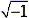. Then we can think of i2 as -1. In general, if c is any positive number, we would write: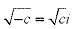.

If we have a complex number z, where z=a+bi then a would be the real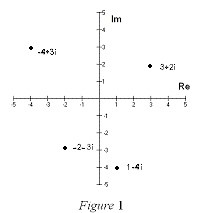component (denoted: Re z) and b would represent the imaginary component of z (denoted Im z). Thus the real component of z=4+3i is 4 and the imaginary component would be 3. From this, it is obvious that two complex numbers (a+bi) and (c+di) are equal if and only if a=c and b=d, that is, the real and imaginary components are equal.

The complex number (a+bi) can also be represented by the ordered pair (a,b) and plotted on a special plane called the complex plane or the Argand Plane. On the Argand Plane the horizontal axis is called the real axis and the vertical axis is called the imaginary axis. This is shown in Figure 1 on the right:

# Properties of the Complex Set

The set of complex numbers is denoted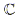. Just like any other number set there are rules of operation.

The sum and difference of complex numbers is defined by adding or subtracting their real components ie: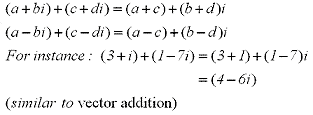The communitive and distributive properties hold for the product of complex numbers ie: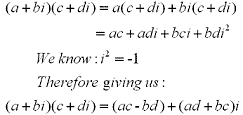When dividing two complex numbers you are basically rationalizing the denominator of a rational expression. If we have a complex number defined as z =a+bi then the conjuate would be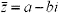. See the following example:

# Example: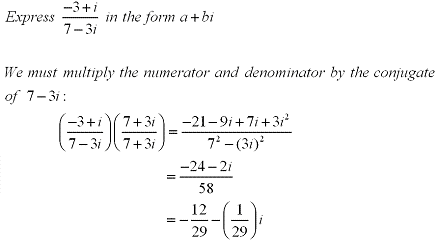# Conjugates

The geometric inperpretation of a complex conjugate is the reflection along the real axis. This can be seen in the figure below where z = a+bi is a complex number. Listed below are also several properties of conjugates.

Properties: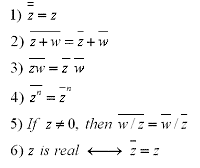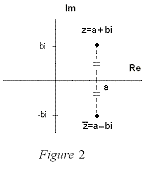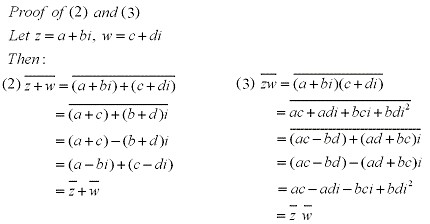# Absolue Value/Modulus

The distance from the origin to any complex number is the absolute value or modulus. Looking at the figure below we can see that Pythagoras' Theorem gives us a formula to calculate the absolute value of a complex number z = a+bi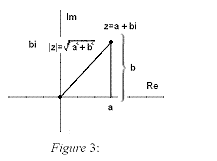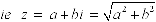And from this we get: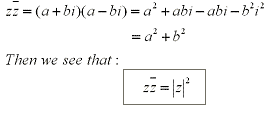This explains why rationalizing the denominator using conjugates works in general for complex quotients.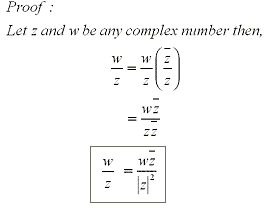There are also some properties of absolute values dealing with complex numbers. These are: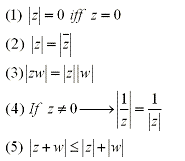# Polar Form

Along with being able to be represented as a point (a,b) on a graph, a complex number z = a+bi can also be represented in polar form as written below: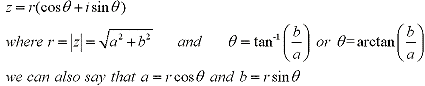Note: The Arg(z) is the angle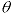, and that this angle is only unique between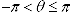which is called the primary angle. Adding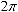# Example:

Using the principle argument, write the following complex number in its polar coordinates.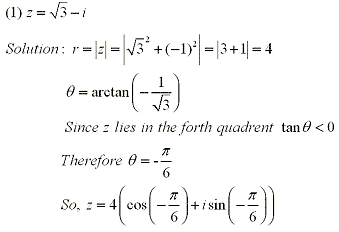Multiplication and division can be given geometric interpretations and new insight when looking at polar forms:

Let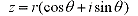and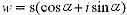be complex numbers.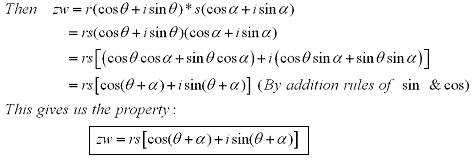We can see from this that in order to multiply two complex numbers we must multiply the length or absolute values together and add the arguments.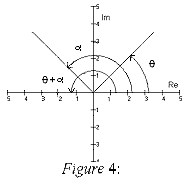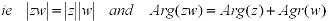In the case of division, it is similarly shown that: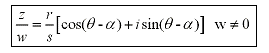by using the subtration rule of sine and cosine, as apose to multipling the lengths we divide and as apose to adding the arguments, we subtract.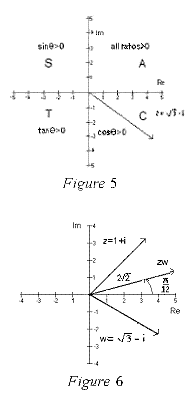# Example: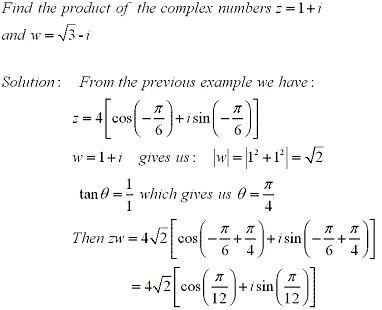# DeMoivre's Theorem

DeMoivre's Theorem is a generalized formula to compute powers of a complex number in it's polar form.

Looking atfrom the eariler formula we can find (z)(z) easily: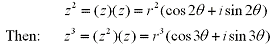Which brings us to DeMoivre's Theorem:
Ifand n are positive integers then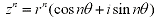Basically, in order to find the nth power of a complex number we take the nth power of the absolute value or length and multiply the argument by n.

# Example: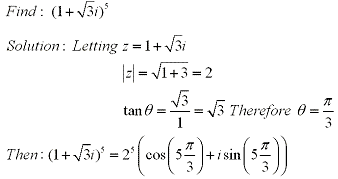Working backwards we can also use DeMoivre's Theorem to find the nth root. Letand n be a positive integer.Then z has n distinct nth roots given by: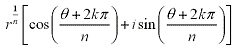where k = 0, 1, 2, ... , n-1

To show this we letandwhere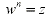.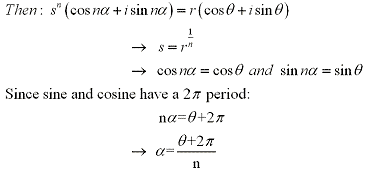# Example: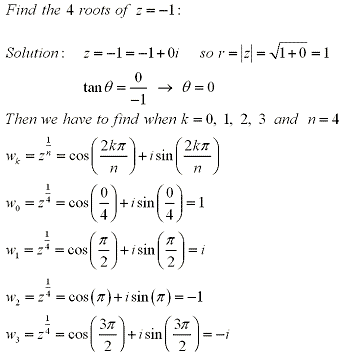# Euler's Fomula

From Calculus, we know the functions ex, sin x and cos x have power series expansions or Taylor series.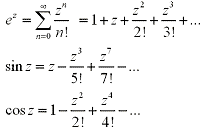When looking at complex numbers it is interesting to see that the power series for ez allows z to be complex and obeys the usual rules for exponents. In particular it holds true that:

ez1+z2 = ez1z2
letting z = iy where i is imaginary and y is a real number

From the fact that: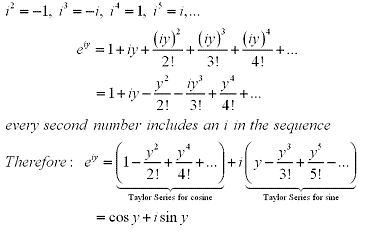This gives us the result of Euler's Formula

eiy = cos y + isin y

Then we can also define z as: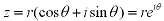# Example: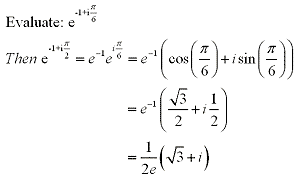It is also important to mention that ifand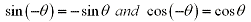From tringonometry we can then say: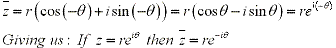NOTE: This website has been designed for and tested for functionality on Internet Explorer 6.0, Netscape Navigator 7.2, Netscape Browser 8.0, and Mozilla Firefox 1.0. Other browsers may not display information correctly, although future versions of the abovementioned browsers should function properly.

COURSE HOMEPAGE
MATH 1046

FACULTY HOMEPAGES
Alex Karassev
Ted Chase